Home > ACC7 > Chapter cc29 > Lesson cc29.2.2 > Problem9-69

9-69.
1. Simplify each of the following expressions. Homework Help ✎

1. 3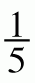·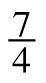2. 53 ·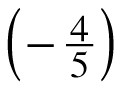3. 24 ·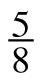4.· 32

5.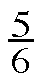+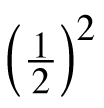6.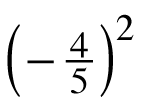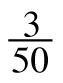7.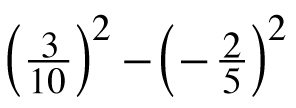8. 82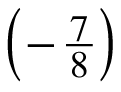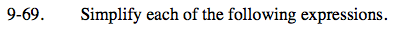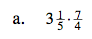Convert the mixed number into a fraction greater than one.

$\frac{16}{5}\left(\frac{7}{4}\right)$

$\frac{28}{5}=5\frac{3}{5}$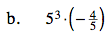$- \frac{4}{5} = (-1)(4)\left(\frac{1}{5}\right)$

Notice that the 5 in the denominator is like dividing by 5.

5(3) /5 (−4)

52(−4) = −100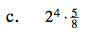This can be rewritten as:

$2^{4}\left(\frac{5}{2^{3}}\right)$

See part (b).

10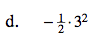Use the order of operations.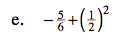Use the order of operations.

$-\frac{5}{6}+\frac{1}{4}$

$-\frac{20}{24}+\frac{6}{24}=-\frac{14}{24}$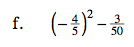Notice that squaring a negative number has the same result as multiplying two negative numbers: the product is always positive.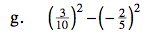Follow the order of operations.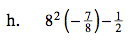$8^{(2-1)}(-7)-\frac{1}{2}$

$8(-7)-\frac{1}{2}$

$(-56)-\frac{1}{2}$

$-56\frac{1}{2}$